﻿ 大口径拼接镜平移误差对其成像质量影响研究 Study on the Influence of Translation Error of Large Aperture Stitching Mirror on Its Imaging Quality

Optoelectronics
Vol. 09  No. 01 ( 2019 ), Article ID: 29445 , 6 pages
10.12677/OE.2019.91009

Study on the Influence of Translation Error of Large Aperture Stitching Mirror on Its Imaging Quality

Xuewei Chen

School of Science, Changchun University of Technology, Changchun Jilin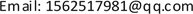Received: Mar. 6th, 2019; accepted: Mar. 19th, 2019; published: Mar. 27th, 2019ABSTRACT

As one of the development trends of larger aperture astronomical telescopes, the common phase errors among the sub-mirrors of the mosaic mirror have a great impact on the image quality of the mosaic mirror. In this paper, the effect of piston error, one of common phase errors, on image quality of stitching mirror is analyzed theoretically, and the effect of translation error on point spread function of stitching mirror system under different wavelengths is simulated and analyzed.

Keywords:Piston Error, Point Spread Function, Space Telescope, Co-Phasing1. 引言

2. 平移误差对拼接镜成像质量影响理论分析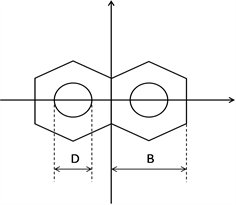Figure 1. Structure of segmented telescope

$U\left(x,y\right)=A\left(x,y\right)\left[circ\frac{x-\frac{B}{2}}{\frac{D}{2}}\ast {\text{e}}^{i{\varphi }_{1}}+circ\frac{x+\frac{B}{2}}{\frac{D}{2}}\ast {\text{e}}^{i{\varphi }_{2}}\right]$ (1)

$A\left(x,y\right)=\left\{\begin{array}{ll}1,\hfill & 子孔内径\hfill \\ 0,\hfill & 子孔径外\hfill \end{array}$ (2)

$\Delta \varphi ={\varphi }_{2}-{\varphi }_{1}=\frac{2\pi }{\lambda }p$ (3)

$PSF\left(u,v,\lambda \right)={|FT\left[U\left(x,y\right)\right]|}^{2}=2\frac{{D}^{2}/2{J}_{1}^{2}\left(\pi D\sqrt{{u}^{2}+{v}^{2}}\right)}{{u}^{2}+{v}^{2}}$ (4)

$PSF\left(x,y,\lambda \right)=2\frac{{D}^{2}/2{f}^{2}{\lambda }^{2}{J}_{1}{}^{2}\left(\pi D/\lambda f\sqrt{{x}^{2}+{y}^{2}}\right)}{{x}^{2}+{y}^{2}}\left[1+\mathrm{cos}\left(\frac{2\pi p}{\lambda }-\frac{2\pi B}{\lambda f}x\right)\right]$ (5)

$PS{F}_{t}\left(u,v,\lambda \right)={\sum }_{k=1}^{n}\frac{PSF\left(u,v,{\lambda }_{k}\right)\Delta \lambda }{n}$ (6)

3. 平移误差对拼接镜成像质量影响仿真分析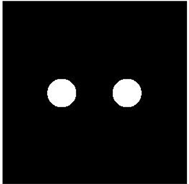(a)(b)

Figure 2. Simulated double pupil structure. (a) Hexagon structure of; (b) Segmented telescope with mask segmented telescope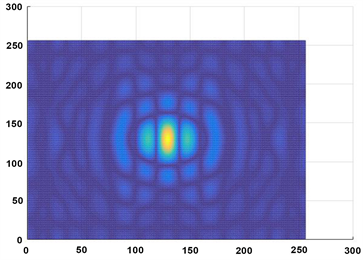(a)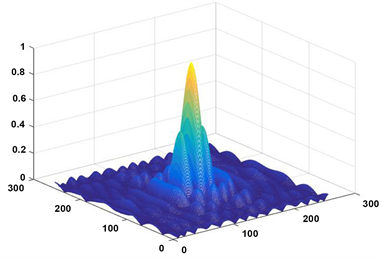(b)

Figure 3. PSF and far field facula at piston error equals 0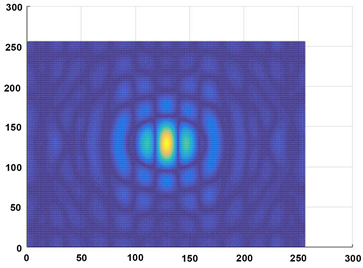(a)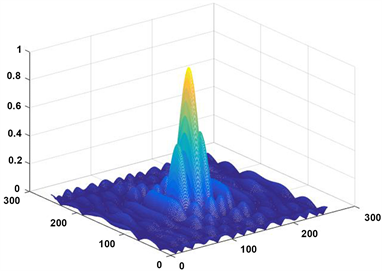(b)

Figure 4. PSF and far field facula at piston error equals λ/16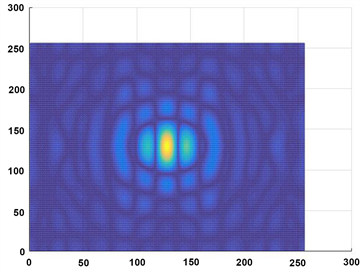(a)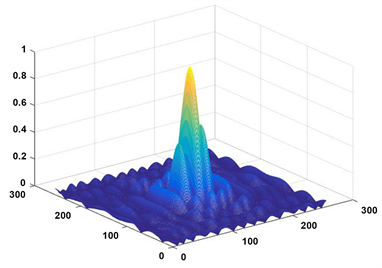(b)

Figure 5. PSF and far field facula at piston error equals λ/8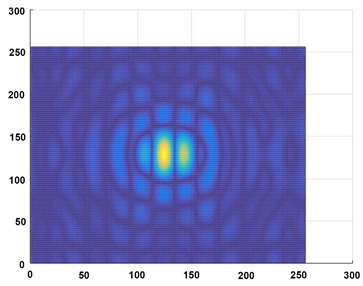(a)(b)

Figure 6. PSF and far field facula at piston error equals λ/4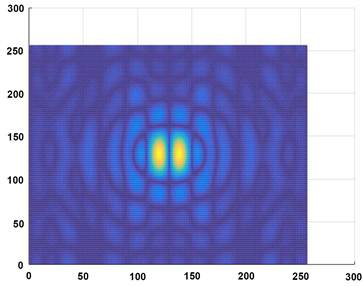(a)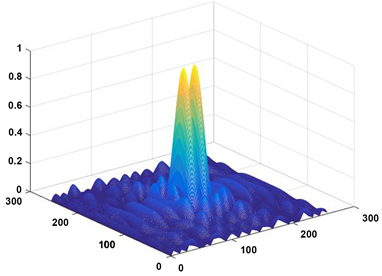(b)

Figure 7. PSF and far field facula at piston error equals λ/2

4. 结论

Study on the Influence of Translation Error of Large Aperture Stitching Mirror on Its Imaging Quality[J]. 光电子, 2019, 09(01): 56-61. https://doi.org/10.12677/OE.2019.91009

1. 1. 王金, 鲜浩, 王胜千, 等. 拼接式望远镜子镜间平移、倾斜误差及子镜间隙对成像质量的影响[J]. 光电工程, 2014(10): 55-62.

2. 2. Lamb, M., Correia, C., Sauvage, J.F., et al. (2017) Quantifying Telescope Phase Discontinuities External to AO-Systems by Use of Phase Diversity and Focal Plane Sharpening. Journal of Astronomical Telescopes, Instruments, and Systems, 3, Article ID: 039001.

3. 3. 卞毓麟. 光学天文望远镜的足迹[J]. 物理, 2008, 37(12): 844-852.

4. 4. 薛艳杰, 薛随建, 朱明, 等. 天文望远镜技术发展现状及对我国未来发展的思考[J]. 中国科学院院刊, 2014(3): 368-375.

5. 5. 杨李成, 凌宁. 发展中的能动薄镜技术[J]. 激光与光电子进展, 2002, 39(2): 10-14.

6. 6. Kendrick, S.E. and Brown, R.J. (2003) Lightweighted Beryllium Cryogenic Mirrors for Both Monolithic and Segmented Space Telescope. Proceedings of SPIE—The International Society for Optical Engineering, Waikoloa, 241-253.

7. 7. Schipani, P., Perrortta, F. and Marty, L. (2006) Active Optics Correction Forces for the VST 2.6M Primary Mirror. Proceedings of SPIE, Orlando, Vol. 6273, 62733A. https://doi.org/10.1117/12.670532

8. 8. Bely, P.Y. (2003) The Design and Construction of Large Optical Telescopes. Springer Science & Business Media, Berlin.

9. 9. 王珊珊, 朱秋东, 曹根瑞. 空间拼接主镜望远镜共相位检测方法[J]. 光学学报, 2009, 29(9): 2435-2440.

10. 10. Chanan, G., Troy, M., Dekens, F., et al. (1998) Phasing the Mirror Segments of the Keck Telescopes: The Broadband Phasing Algorithm. Applied Optics, 37, 4706-4714. https://doi.org/10.1364/AO.37.000140